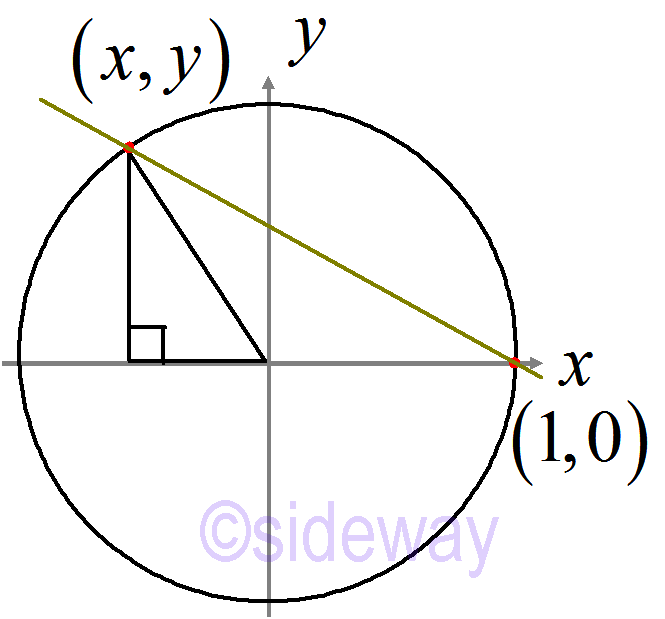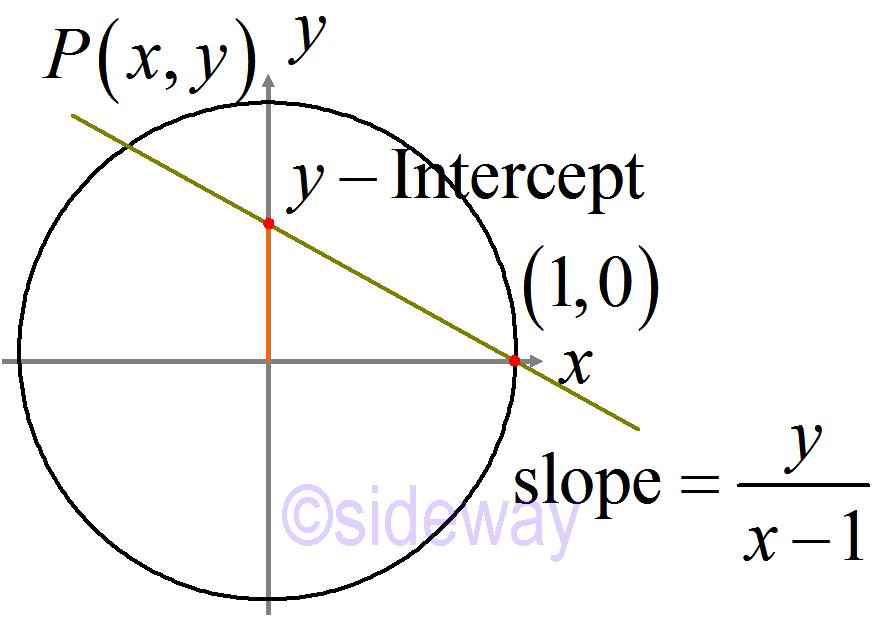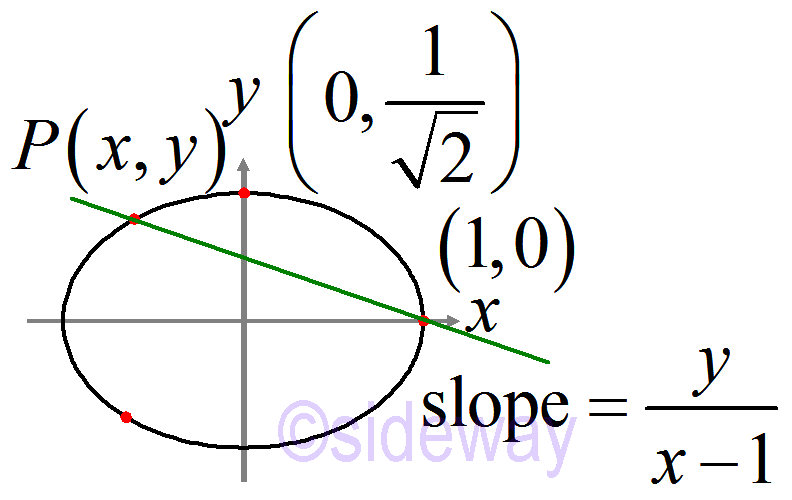output.to from Sideway
Draft for Information Only

# Content

` Pythagorean Triples Geometric Approach 𝑦-intercept Conic Section Example 𝑥2+2𝑦2=1 Key Ingredients for Binary Operation: 𝑥2+𝑑𝑦2=1  Generalization to degree 3 with three variables  Generalization to twists: 𝑎𝑥2+𝑏𝑦2=𝑐→𝑥2+𝑎𝑦2=𝑏 Key Ingredients for Parameterisation: 𝑥2+𝑑𝑦2=1 Source and Reference`

## Pythagorean TriplesPythagorean triples: 𝑥2+𝑦2=1 with rational solutions. The rational solution (𝑥,𝑦) can also be reference to another fixed rational point i.e. (1,0)

## Geometric ApproachBy sweeping a line about a fixed rational point (1,0), the slope of the line jointing the rational point (𝑥,𝑦) can be used to represent this rational point. And the slope is equal to 𝑦𝑥−1. Since the rational solutions of 𝑥2+𝑦2=1 always preserves the rationality of slope. In other words, the rational point (𝑥,𝑦) is mapped to a rational slope. ```𝑍0={(𝑥,𝑦):𝑥2+𝑦2=1,𝑥≠1} 𝑚:𝑍0→ℚ given by 𝑚(𝑃)=𝑦𝑥−1 ``` Similarly, the map 𝑚 can be defined for more general curves. And the properties of 𝑚 are
• 𝑚 is 1-to-1
• 𝑚 is surjective.
• 𝑚−1:ℚ→𝑍0 is given by rational functions
These properties⇒a parameterisation

## 𝑦-interceptSimilar to Riemann stereographic projection, the slope of the sweeping line can be projected as the 𝑦-intercept on the 𝑦-axis. The parameterisation of the 2-degee equation with one fixed point gives one unique association with the remaining point. Let 𝑚−1(𝑡)=(𝑥,𝑦); 𝑥2+𝑦2=1 ⇒𝑚(𝑃)=𝑡⇒𝑦𝑥−1=𝑡 ⇒𝑦=𝑡(𝑥−1) and 𝑥2+𝑦2=1 Let 𝑧=𝑥−1 ⇒𝑦=𝑡𝑧 and (𝑧+1)2+𝑦2=1 ⇒(𝑧+1)2+(𝑡𝑧)2=1 ⇒𝑧2+2𝑧+𝑡2𝑧2=0 ⇒(1+𝑡2)𝑧2+2𝑧=0 If 𝑧≠0 ⇒(1+𝑡2)𝑧+𝑧=0 ⇒𝑧=−21+𝑡2⇒𝑥−1=−21+𝑡2 ⇒𝑥=𝑡2−11+𝑡2∊ℚ ⇒𝑦=𝑡𝑧=𝑡21+𝑡2=−2𝑡1+𝑡2 ⇒𝑚−1(𝑡)=(𝑥,𝑦)=𝑡2−11+𝑡2,−2𝑡1+𝑡2

## Conic Section Example 𝑥2+2𝑦2=1

Similar to other conic section.Binary Operation of 𝑥2+2𝑦2=1
Let 𝑃(𝑥,𝑦),𝑄(𝑤,𝑧) be the solution of the equation. ⇒(𝑥+𝑦2𝑖)(𝑥−𝑦2𝑖)=1 and (𝑤+𝑧2𝑖)(𝑤−𝑧2𝑖)=1 ⇒(𝑥𝑧−2𝑦𝑤)2+2(𝑥𝑤+𝑦𝑧)2=1 So 𝑃⊕𝑄=𝑅 where 𝑅=(𝑥𝑧−2𝑦𝑤,𝑥𝑤+𝑦𝑧)map 𝑚:𝑍0→ℚ given by 𝑚(𝑃)=𝑦𝑥−1 where 𝑍0={(𝑥,𝑦):𝑥2+2𝑦2=1, 𝑥≠1} 𝑚−1(𝑡)=(𝑥,𝑦), where 𝑦=𝑡(𝑥−1) Let 𝑧=𝑥−1⇒(𝑧+1)2+2𝑡2𝑧2=1⇒𝑧((1+2𝑡2)𝑧+2)=0 ⇒𝑧=−2/(1+2𝑡2) ⇒𝑥=2𝑡2−11+2𝑡2 ⇒𝑦=−2𝑡21+2𝑡2 ⇒𝑚−1(𝑡)=2𝑡2−11+2𝑡2,−2𝑡21+2𝑡2 𝑚−1:ℚ→𝑍0

## Key Ingredients for Binary Operation: 𝑥2+𝑑𝑦2=1

𝑥2+𝑑𝑦2=1
• RHS being 1
• (𝑥+𝑦𝑑)𝑛=𝑥'+𝑦'𝑑⇒𝑥2𝑛+𝑑𝑦2𝑛=1

### Generalization to degree 3 with three variables

𝑥3+2𝑦3−6𝑥𝑦𝑧+4𝑧3=1⇒manipulate 2

## Key Ingredients for Parameterisation: 𝑥2+𝑑𝑦2=1

• A rational point (1,0)
• The degree being 2⇒𝑚−1:ℚ→𝑍0

## Source and Reference

ID: 201100016 Last Updated: 11/16/2020 Revision: 0 Ref:References

1. B. Joseph, 1978, University Mathematics: A Textbook for Students of Science &amp; Engineering
2. Wheatstone, C., 1854, On the Formation of Powers from Arithmetical Progressions
3. Stroud, K.A., 2001, Engineering Mathematics
4. Coolidge, J.L., 1949, The Story of The Binomial TheoremHome 5

Management

HBR 3

Information

Recreation

Culture

Chinese 1097

English 337

Computer

Hardware 153

Software

Application 206

Latex 35

Manim 172

Numeric 19

Programming

Web 285

Unicode 504

HTML 65

CSS 59

ASP.NET 196

OS 422

Python 61

Knowledge

Mathematics

Algebra 31

Geometry 21

Calculus 67

Engineering

Mechanical

Rigid Bodies

Statics 92

Dynamics 37

Control

Natural Sciences

Electric 27